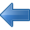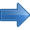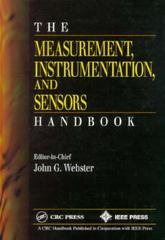Electrostatic Applications Books by Topic Author IndexPrevious Laboratory Work Next# The Measurement, Instrumentation and Sensors Handbook

## John G. Webster, ed.

1999

2608 pages

The Measurement Instrumentation and Sensors Handbook describes the use of instruments and techniques for practical measurements required in engineering, physics, chemistry, and the life sciences. The book examines:

Organized according to the measurement problem, each section addresses the different ways of making a measurement for a given variable. Chapters present information on three levels:

The Measurement, Instrumentation and Sensors Handbook provides a graded level of difficulty from start to finish, serving the reference needs of the broadest group of readers. Edited by one of the more noted instrumentation experts in the world, the book contains nearly 150 contributions, covering all aspects on the design and implementation of various instrumentation.

"…concise and useful reference covering a wide range of measurement, instrumentation, and sensor topics…With 14 chapters filled with a wealth of useful information, this comprehensive reference will be a valuable addition to anyone who works with instrumentation and measurements."
--John J. Shea, IEEE Electrical Insulation Magazine, Vol. 16, No. 4

Contents

Measurement Characteristics
Characteristics of Instrumentation
Operational Modes of Instrumentation
Static and Dynamic Characteristics of Instrumentation
Measurement Accuracy
Measurement Standards
Spatial Variables Measurement
Displacement, Linear and Angular
Thickness Measurement
Proximity Measurement
Distance Measurement
Position, Location, Attitude Measurement
Level Measurement
Area Measurement
Volume Measurement
Angle Measurement
Tilt Measurement
Velocity Measurement
Acceleration, Vibration and Shock Measurement
Time and Frequency Measurement
Time Measurement
Frequency Measurement
Mechanical Variables Measurement - Solid
Mass and Weight Measurement
Density Measurement
Hardness Measurement
Stress Measurement
Strain Measurement
Force Measurement
Torque and Power Measurement
Elastic Moduli and Stiffness Measurement
Tactile Sensing Measurement
Mechanical Variables Measurement - Fluid
Pressure and Sound Measurement
Acoustic Measurement
Flow Measurement
Point Velocity Measurement
Viscosity Measurement
Surface Tension Measurement
Mechanical Variables - Thermal
Temperature Measurement
Thermal Conductivity Measurement
Heat Flux Measurement
Thermal Imaging
Calorimetry Measurement
Electromagnetic Variables Measurement
Voltage Measurement
Current Measurement
Power Measurement
Power Factor Measurement
Phase Measurement
Energy Measurement
Conductivity and Resistivity Measurement
Charge Measurement
Capacitance Measurement
Permittivity Measurement
Electric Field Measurement
Magnetic Field Measurement
Permeability and Hysteresis Measurement
Inductance Measurement
Immittance Measurement
Q-Factor Measurement
Attenuation Measurement
Distortion Measurement
Noise Measurement
Intermodulation Measurement
Microwave Measurement
Optical Variables Measurement
Densitometry Measurement
Colorimetry Measurement
Optical Loss Measurement
Polarization Measurement
Refractive Index Measurement
Turbidimetry Measurement
Laser Measurement
Vision and Image Sensors
X-Ray/Gamma Ray Measurement
Charged Particle Measurement
Neutron Measurement
Dosimetry Measurement
Chemical Variables Measurement
Composition Measurement
pH Measurement
Humidity and Moisture Measurement
Environmental Measurement
Biomedical Variables Measurement
Biopotentials and Electrophysiology Measurement
Blood Pressure Measurement
Blood Flow Measurement
Ventilation Measurement
Blood Chemistry Measurement
Medical Imaging Measurement
Signal Processing
Amplifiers and Signal Conditioners
Modulation
Filters
Spectrum Analysis and Correlation
Analog-to-Digital Converters
Computers
Telemetry
Sensor Networks and Communication
Electromagnetic Compatibility
Displays
Human Factors in Displays
Cathode-Ray Tube Displays
Liquid Crystal Displays
Plasma Displays
Electroluminescent Displays
Light-Emitting Diode Displays
Control
PID Control
Optimal Control
Electropneumatic and Electrohydraulic Instruments
Explosion-Proof Instruments
Positioning and Motion Control
Appendices
Conversion Factors (English to SI; SI Prefixes)
Index